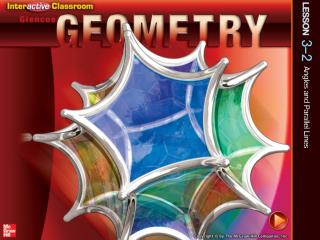Download PresentationSplash Screen# Splash Screen - PowerPoint PPT Presentation

Download Presentation##### Splash Screen

Download Policy: Content on the Website is provided to you AS IS for your information and personal use and may not be sold / licensed / shared on other websites without getting consent from its author. While downloading, if for some reason you are not able to download a presentation, the publisher may have deleted the file from their server.

- - - - - - - - - - - - - - - - - - - - - - - - - - - E N D - - - - - - - - - - - - - - - - - - - - - - - - - - -
##### Presentation Transcript

1. Splash Screen

2. Five-Minute Check (over Lesson 3–1) CCSS Then/Now Postulate 3.1: Corresponding Angles Postulate Example 1: Use Corresponding Angles Postulate Theorems: Parallel Lines and Angle Pairs Proof: Alternate Interior Angles Theorem Example 2: Real-World Example: Use Theorems about Parallel Lines Example 3: Find Values of Variables Theorem 3.4: Perpendicular Transversal Theorem Lesson Menu

3. Choose the plane parallel to plane MNR. A.RST B.PON C.STQ D.POS 5-Minute Check 1

4. Choose the segment skew to MP. ___ A.PM B.TS C.PO D.MQ ___ ___ ___ 5-Minute Check 2

5. Classify the relationship between 1 and 5. A. corresponding angles B. vertical angles C. consecutive interior angles D. alternate exterior angles 5-Minute Check 3

6. Classify the relationship between 3 and 8. A. alternate interior angles B. alternate exterior angles C. corresponding angles D. consecutive interior angles 5-Minute Check 4

7. Classify the relationship between 4 and 6. A. alternate interior angles B. alternate exterior angles C. corresponding angles D. vertical angles 5-Minute Check 5

8. Which of the following segmentsis not parallel to PT? A.OS B.TS C.NR D.MQ 5-Minute Check 6

9. Content Standards G.CO.1 Know precise definitions of angle, circle, perpendicular line, parallel line, and line segment, based on the undefined notions of point, line, distance along a line, and distance around a circular arc. G.CO.9 Prove theorems about lines and angles. Mathematical Practices 1 Make sense of problems and persevere in solving them. 3 Construct viable arguments and critique the reasoning of others. CCSS

10. You named angle pairs formed by parallel lines and transversals. • Use theorems to determine the relationships between specific pairs of angles. • Use algebra to find angle measurements. Then/Now

11. Concept

12. Use Corresponding Angles Postulate A. In the figure, m11 = 51. Find m15. Tell which postulates (or theorems) you used. 15  11 Corresponding Angles Postulate m15 =m11 Definition of congruent angles m15 =51 Substitution Answer: m15 = 51 Example 1

13. Use Corresponding Angles Postulate B. In the figure, m11 = 51. Find m16. Tell which postulates (or theorems) you used. 16  15 Vertical Angles Theorem 15 11 Corresponding Angles Postulate 16 11 Transitive Property () m16 = m11 Definition of congruent angles m16 = 51 Substitution Answer: m16 = 51 Example 1

14. A. In the figure, a || b and m18 = 42. Find m22. A. 42 B. 84 C. 48 D. 138 Example 1a

15. B. In the figure, a || b and m18 = 42. Find m25. A. 42 B. 84 C. 48 D. 138 Example 1b

16. Concept

17. Concept

18. Use Theorems about Parallel Lines FLOOR TILES The diagram represents the floor tiles in Michelle’s house. If m2 = 125, find m3. 2  3 Alternate Interior Angles Theorem m2 =m3 Definition of congruent angles 125 =m3 Substitution Answer:m3 = 125 Example 2

19. FLOOR TILES The diagram represents the floor tiles in Michelle’s house. If m2 = 125, find m4. A. 25 B. 55 C. 70 D. 125 Example 2

20. Find Values of Variables A. ALGEBRA If m5 = 2x – 10, and m7 = x + 15, find x. 5  7 Corresponding Angles Postulate m5 =m7 Definition of congruent angles 2x – 10 =x + 15 Substitution x – 10= 15 Subtract x from each side. x = 25 Add 10 to each side. Answer:x = 25 Example 3

21. Find Values of Variables B. ALGEBRA If m4 = 4(y – 25), and m8 = 4y, find y. 8  6 Corresponding Angles Postulate m8 = m6 Definition of congruent angles 4y = m6 Substitution Example 3

22. Find Values of Variables m6 + m4 = 180 Supplement Theorem 4y + 4(y – 25) = 180 Substitution 4y + 4y – 100 = 180 Distributive Property 8y = 280 Add 100 to each side. y = 35 Divide each side by 8. Answer:y = 35 Example 3

23. A. ALGEBRA If m1 = 9x + 6, m2 = 2(5x – 3), and m3 = 5y + 14, find x. A.x = 9 B.x = 12 C.x = 10 D.x = 14 Example 3

24. B. ALGEBRA If m1 = 9x + 6, m2 = 2(5x – 3), and m3 = 5y + 14, find y. A.y = 14 B.y = 20 C.y = 16 D.y = 24 Example 3

25. Concept

26. End of the Lesson